# Resonant Modes of a Conical Cavity

## by Greg EganEnergy-momentum conservation has been a cornerstone of physics for more than a century. Nowhere does its essence shine forth so clearly as in Einstein’s geometric formulation of it.

— Charles Misner, Kip Thorne and John Wheeler, Gravitation

If we are willing parties, scientific controversies can be conjured at the drop of a hat. We must all share the responsibility of separating real scientific controversies from fake ones or we will unwittingly create the conditions for a technological populism we will live to regret.

— Harry Collins, New Scientist, 17 March 2007, p 55.

In September 2006, the magazine New Scientist published an unfortunate beat-up  in which they hyped the immensely unlikely claims of a British engineer named Roger Shawyer, who had designed what he described as an “electromagnetic drive” (aka “EmDrive”) for satellites. This device supposedly produced a net thrust solely by bouncing microwaves back and forth in a closed metal cavity, the idea being that making the cavity asymmetric would mean that a greater force would be exerted by the microwaves on one end compared to the other, causing the entire device to accelerate .

[Eight years later, in 2014, sections of the media are now giving the same kind of beat-up to equally unlikely claims from a team of NASA engineers, although this time the rationale for the device has shifted from classical electromagnetism to a misunderstanding of the quantum vacuum. None of the experiments that are claimed to support the idea that closed cavities like this can generate thrust have come close to the necessary degree of rigour needed to account for the forces produced by (a) thermally driven air currents (which will depend on the detailed surface temperature distribution of the cavity, as well as its precise geometry) and (b) conventional electromagnetic forces between the device being tested and the surrounding equipment.]

It’s obvious from conservation of momentum that such a drive can’t work. Because it doesn’t emit anything (not even microwaves), if it caused a spacecraft to accelerate there would be nothing to balance the change in the spacecraft’s momentum. Shawyer claimed that the microwaves can somehow balance the spacecraft’s momentum while remaining inside the cavity , but has offered no coherent explanation for how this happens, nor how momentum can be conserved when the engine has been shut off, the microwaves have been absorbed by the cavity walls, and the spacecraft is still moving differently than it was initially.

I’ve contributed to some blog discussions [4,5,6] on New Scientist’s falling standards and other aspects of this matter, but the purpose of this web page is to address one very specific issue. Although it’s clear from basic principles that no closed microwave cavity can experience a net thrust due to the radiation pressure from its contents, it’s of some interest to perform a detailed analysis of an asymmetric cavity broadly similar to Shawyer’s design, and to calculate the distribution of forces on the walls.

Shawyer’s design  appears to be a truncated cone, with a simple flat metal cap at the broad end and a small dielectric-filled cylinder attached to the narrow end. To simplify things, I’ve analysed a “truncated spherical cone”, i.e. a cavity whose side walls are exactly the same as an ordinary truncated cone, but whose end caps are portions of spheres whose centres both lie at the apex that the cone would reach were it not truncated. This allows the problem to be treated exactly in spherical polar coordinates, with all three boundaries being surfaces with constant values for one or other of the coordinates.

I should stress that this analysis is not necessary in order to prove that the theoretical basis of Shawyer’s drive is false. Any calculation correctly derived from Maxwell’s equations of electromagnetism coupled with matter (whether the matter is treated with Newtonian mechanics, or with relativistic mechanics) will necessarily respect conservation of momentum, whereas it is plain that Shawyer’s claims for his drive do not respect that principle.

However, though it’s clear that the net forces must sum to zero, it’s still fun to work out the details and see exactly how this happens. There’s even a small surprise: for some modes, parts of the cavity wall are under tension, not pressure! To see why, read on ...

(Readers who find the mathematics here a bit heavy going might have a look at this simpler example of a two-dimensional asymmetric resonant cavity.)

## Field solutions

Our main goal is to compute the force on the walls of a resonant cavity, due to the electromagnetic field within the cavity. An electromagnetic field exerts pressure on neutral matter that is equal to the density of the energy stored in the field. But we will need to be careful, because some modes of the cavity radiation will induce a redistribution of electric charge in the cavity walls, and there will be an additional force due to the interaction of the electric field and that charge distribution.

We will treat the interior of the cavity as a vacuum, so within it the electric field E(t) and the magnetic field B(t) will both obey the vector wave equation:

2F(t) = (1/c2) ∂2F(t) / ∂t2

where we will use the vector F to stand for either E or B. Because we are interested in resonant modes of the cavity, we will be looking for solutions of the wave equation that represent standing waves. These solutions will behave harmonically with time, i.e. the time variation of each will be entirely described by a multiplicative factor of the form sin(ωt) or cos(ωt). In a standing wave E and B will be 90 degrees out of phase, and we will choose the origin for t such that:

E(t) = E sin(ωt)
B(t) = B cos(ωt)

where we’re writing E and B to represent the time-independent spatial patterns for the vector fields. These time-independent fields must then satisfy the vector Helmholtz equation:

2F + k2 F = 0, where k = ω/cOne boundary condition that we will need to impose is that the electric field E at each cavity wall can not have a component parallel to it; it must be entirely orthogonal to the wall, or it can be zero there. If the electric field did have a component parallel to the wall, it would cause charges to flow within the wall until they produced their own field that cancelled it out.

The magnetic field B, in contrast, can not have a component orthogonal to the wall; it must either be zero there, or lie parallel to the wall. This is because our magnetic field is oscillating; the actual field from moment to moment is B(t) = B cos(ωt). A time-varying magnetic field is linked to the electric field by one of Maxwell’s equations: curl E = –∂B/∂t. So an oscillating magnetic field with a component orthogonal to the wall would induce circular currents in the wall that would cancel out the field.

In order to meet these conditions, we’ll need to work in a coordinate system in which the cavity walls have a simple description. We will position the truncated spherical cone so that its axis lies on the z-axis of our coordinate system, its would-be apex lies at the origin, and in polar coordinates (r, θ, φ) — where r is the distance from the origin, θ is the angle from the z-axis, and φ is the angle from the xz plane — the side walls of the cone will be described by θ = θw, its narrow end will be described by r = r1, and its wide end will be described by r = r2. We will write {er, eθ, eφ} for the unit vectors in each of the coordinate directions at any given point.

We will seek solutions of the vector Helmholtz equation that comply with these boundary conditions. As a first step, we note that a complete set of solutions of the scalar Helmholtz equation (where the vector F is replaced by a scalar function f) consisting of products of functions of the three spherical polar coordinates, is given by :

f(r, θ, φ) = R±(r) Q(θ) F(φ)
R±(r) = (1/√r) J±(n+1/2)(k r)
Q(θ) = P|m|n(cos θ)
F(φ) = cos(mφ)

where J is a Bessel function, and P is an associated Legendre function. To ensure continuity at φ = 0 with φ = 2π, the parameter m must be an integer; it can be negative, positive or zero. The allowed values of n will be related to the boundary conditions; we will have more to say on this shortly.

How does it help us to have solutions to the scalar equation? It turns out that there’s a nice trick for generating vector solutions from scalar solutions . Given a scalar solution f, the vector fields:

M = curl(r f er)
N = curl curl(r f er)

will both solve the vector Helmholtz equation. (Note that although  also gives the vector field L = grad f as a further solution, the curl of a gradient is zero, and if either curl E = 0 or curl B = 0 that would imply, via Maxwell’s equations, a completely static solution.)

If f is taken from our set of scalar solutions of the Helmholtz equation, we then have for M :

M = R±(r) [ (Q(θ) / sin θ) F '(φ) eθQ'(θ) F(φ) eφ ]
F '(φ) = –m sin(mφ)
Q'(θ) = [n cos θ P|m|n(cos θ) – (|m|+n) P|m|n–1(cos θ)] / sin θ

The vector field M has no radial component, which means that at the end caps of the cone it will have no component orthogonal to the cavity walls. That’s the boundary condition that must be satisfied by the magnetic field, B, so let’s take M as a possible solution for B.

To simplify things, we’ll confine the remainder of the analysis to modes with m = 0, that is, modes which have no dependence on φ, and hence are rotationally symmetric around the z-axis. We then have:

B = –R±(r) Q'(θ) eφ
Q'(θ) = n [cos θ Pn(cos θ) – Pn–1(cos θ)] / sin θ

This field now automatically fulfils the boundary conditions required of the magnetic field at the cone’s side walls, since it has no component orthogonal to the side walls.

Now, Maxwell’s equations in a vacuum give us:

(1/c2) ∂E(t) / ∂t = curl B(t)

from which it follows, given the harmonic time variation of the fields, that:

E = (c2/ω) curl B = (c2/ω) [(1/r) R±(r) Q1(θ) er + R±1(r) Q'(θ) eθ ]
Q1(θ) = – (Q''(θ) + cot θ Q'(θ)) = n(n+1) Q(θ)
R±1(r) = R±(r)/r + R±'(r) = (1/2) [1/(√r3) J±(n+1/2)(k r) ± k/(√r) (J±(n–1/2)(k r) – J±(n+3/2)(k r))]

The simplification of Q1(θ) to a multiple of Q(θ) follows directly from the differential equation satisfied by Legendre functions.

To meet the boundary conditions for the electric field E on the side wall, we need the radial component of the field to vanish, and hence Q1w) = 0. There will be an infinite set of discrete (but non-integer) values for n that meet this condition, with a choice as to the number of zeroes of Q1 that occur for values of θ smaller than θw. To give a concrete example, suppose that θw = 20°. We can plot Q1w) against n:We see that there are three (non-zero) values of n that satisfy the boundary condition within the range plotted. We can then plot Q1(θ) for these values; in the plot below the three curves are coloured red, green and blue in order of ascending n value.So successive values of n produce increasing numbers of extra zeroes of Q1, as well as the required zero at θw.

Next, we need to meet the boundary conditions for the electric field on the two end caps, where the field’s component in the θ direction must vanish. This is more complicated, because we can’t do it merely by tuning the frequency ω; we also have to mix the two different versions of R±1 that we get by choosing the plus or minus signs in its definition.

We will define:

Rα1(r) = cos α R+1(r) + sin α R1(r)

To demonstrate how the boundary conditions can be met by the right combinations of α and ω, we will choose specific values for the end cap radii: r1 = 2.5, r2 = 10. We then plot the lines where Rα1(r1) = 0 (in red) and Rα1(r2) = 0 (in green), for a fixed value of n (here, the lowest that meets the side wall boundary condition with θw = 20°) over a range of values for α and k; recall that k = ω/c.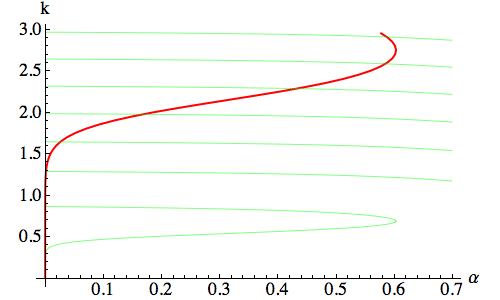Wherever the red and green curves above intersect, we have met the boundary conditions for both of the end caps. (For k < 0.3 the curves are so close that they appear to overlap in this figure, but in fact they don’t intersect.) Below, Rα1(r) is plotted for the three solutions with the lowest k values.As we might have expected, successive values of k produce increasing numbers of extra zeroes of Rα1, in addition to the required zeroes at r1 and r2.

The field modes we have discussed so far, in which the magnetic field B is derived from the M field and the electric field E corresponds to the N field, consist of loops of magnetic flux that circle around the axis of the cone, and an electric field whose direction always lies within a plane passing through the axis. We will refer to these modes as transverse magnetic, or TM modes, since they are quite closely analogous to the modes that go by that name in waveguide theory. Although we are dealing with standing waves and waveguides contain travelling waves, if we think of the axis of the cone as the longitudinal direction, then the magnetic field in these modes is orthogonal, or transverse, to that direction.

Because the electric field in these modes does not vanish as it approaches the cavity walls, it will induce a charge distribution on the inner surface of the metal. Because the electric field is orthogonal to the surface, by Gauss’s Law the charge density σ will be proportional to the magnitude of the electric field:

σ = ε0 E

where ε0 is the permittivity of the vacuum. This charge distribution will then be subject to the electric field, resulting in a Coulomb force that will exert tension on the cavity wall. We will need to take account of this when computing the net force on the wall in the next section.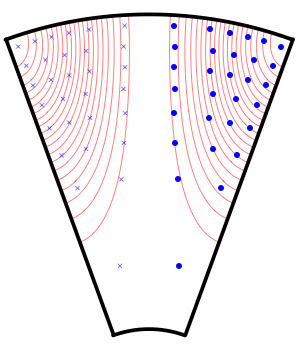Fields for Transverse Magnetic (TM) modes: lowest n value, three lowest k values.

 Numerical data for example TM modes, r1=2.5 cm, r2=10 cm, θw = 20° n = 6.38323 n = 6.38323 n = 6.38323 k = 0.861947 cm–1 k = 1.2847 cm–1 k = 1.6409 cm–1 freq = 4.12 GHz freq = 6.13 GHz freq = 7.83 GHz

We can swap the roles played by M and N and have a solution where the electric field E corresponds to the M field:

E = –R±(r) Q'(θ) eφ

However, the boundary conditions we need to impose are now different. Since this field wraps around the axis in the φ direction, which is not orthogonal to any of the cavity walls, we need to make it vanish completely on all of the walls. So, we need to choose n such that Q'(θw) = 0, and then we need to mix the two different versions of R±:

Rα(r) = cos α R+(r) + sin α R(r)

and choose α and ω so that Rα(r1) = Rα(r2) = 0.

We will give an example for the same cavity geometry used previously: θw = 20°, r1 = 2.5, r2 = 10. Below is a plot of Q'(θw) versus n.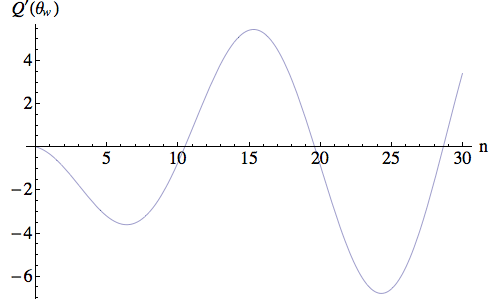The non-zero values of n for which Q'(θw)=0 in the plot above meet the boundary conditions for the side wall, with successive values of n providing extra zeroes of Q', as shown below.If we take the lowest value of n that meets the side wall boundary conditions, we can then make a plot of the values for k and α where each of the end cap boundary conditions are met; values that yield Rα(r1) = 0 are plotted in red, and values that yield Rα(r2) = 0 are plotted in green.Once again, there is a region where these curves come so close that they appear to overlap, but they do not actually intersect there. Finally, we plot Rα(r) for the three lowest k values that satisfy the boundary conditions.The magnetic field, B, can now be found from Maxwell’s equations and the harmonic time variation:

B = (1/ω) curl E = (1/ω) [(1/r) Rα(r) Q1(θ) er + Rα1(r) Q'(θ) eθ ]

Modes of this second kind will have loops of electric flux that circle the axis of the cone, and loops of magnetic flux that lie in planes that pass through the axis. We will refer to these modes as transverse electric, or TE, modes, again in analogy to standard waveguide terminology. Unlike the TM modes, the electric field never makes contact with the cavity wall, so there is no charge distribution and no Coulomb force.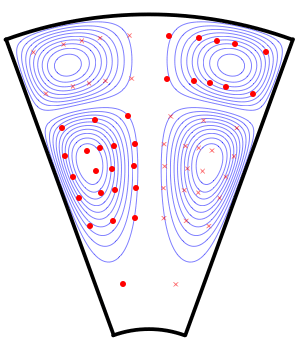Fields for Transverse Electric (TE) modes: lowest n value, three lowest k values.

 Numerical data for example TE modes, r1=2.5 cm, r2=10 cm, θw = 20° n = 10.4885 n = 10.4885 n = 10.4885 k = 1.55771 cm–1 k = 1.96024 cm–1 k = 2.326 cm–1 freq = 7.438 GHz freq = 9.359 GHz freq = 11.10 GHz

## Energy, pressure and forces

The energy density of an electromagnetic field is given by:

u = (1/2) (ε0 E2 + B2 / μ0) = (ε0 / 2) (E2 + c2 B2)

where ε0 and μ0 are the permittivity and permeability of the vacuum (with ε0 μ0 = 1 / c2), and E and B are the magnitudes of E(t) and B(t). If we standardise the phase and the overall normalisation between our two kinds of modes, we can describe their energy density with a single function:

u(t) = U0 [ ([(1/r) Rα(r) Q1(θ)]2 + [Rα1(r) Q'(θ)]2) sin(ωt)2 + k2 [Rα(r) Q'(θ)]2 cos(ωt)2 ]

The constant U0 will set the overall energy in the cavity, though its exact proportionality to the total energy Utotal will be mode-dependent. The simplest way to find Utotal is to set t=0 in the above expression, and then integrate the single non-zero term over the volume of the cavity.

 Numerical data for example modes, r1=2.5 cm, r2=10 cm, θw = 20° TM modes: Utotal / U0 = 7.54728 16.7143 22.0923 TE modes: Utotal / U0 = 26.5626 38.746 49.0616

Below are images of the energy density, averaged over one cycle, on a cross-section through the cavity for the same three TM modes as used in our previous examples. The grey scales are normalised individually, so that white = the maximum energy density in each particular image.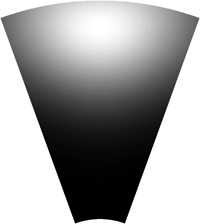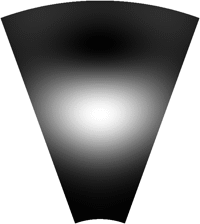Energy density for Transverse Magnetic (TM) modes: lowest n value, three lowest k values.

And these are the energy densities for the corresponding TE modes: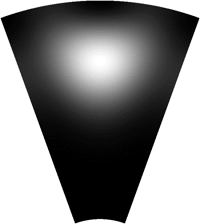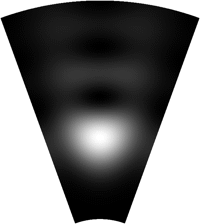Energy density for Transverse Electric (TE) modes: lowest n value, three lowest k values.

The pressure exerted by an electromagnetic field on neutral matter is simply equal to its energy density (the two quantities have the same units), and is uniformly exerted in all directions orthogonal to the lines of magnetic flux. Since the boundary conditions require that the magnetic field is parallel to the cavity walls, the pressure exerted on the walls will be orthogonal to them, and equal to the energy density there.

However, for the TM modes we need to subtract the tension on the walls due to the Coulomb force acting on the charge distribution induced by the electric field meeting the walls. Since the charge density is:

σ = ε0 E

the Coulomb force per unit area, or tension, will be:

T = σ E = ε0 E2

This tension is exactly double the pressure due to the electric field, so the result is that, for the TM modes, we have to reverse the sign of the contribution to the force that is due to the electric field, compared to the formula we’ll use for the TE mode when there is only radiation pressure.

We are interested in the distribution of force on the walls, and it is obvious from the axial symmetry (of both the cavity itself and the fields we’re considering) that if we integrate over a cross-section of the wall taken in a plane orthogonal to the z-axis — i.e. integrate over the φ coordinate — the net force on each such cross-section will be directed solely along the z-axis. To perform this integration, we simply need to account for the area of the cross-section (per coordinate unit, i.e. per unit of r for the side wall, or per unit of θ for the end caps), and the fact that we are taking the z-component of the force. We will also integrate over time, for a full cycle of the mode, to obtain the time-averaged force.

Along the side wall, the area of each cross-section per unit of r is 2π r sin θw, and taking the z-component gives a factor of –sin θw. On the end caps, the area of each cross-section per unit of θ is 2π r1,22 sin θ, and taking the z-component gives a factor of ± cos θ (+ve for the large end cap, -ve for the small one). We will absorb the common factor of 2π, along with a factor of 1/2 due to the integration over time, into a new overall normalisation factor, F0 = πU0.

So, for the TM modes we have:

TM mode, side wall:
F(r) = –F0 [Q'(θw) sin θw]2 (–Rα1(r)2 + k2 Rα(r)2) r
F(r) dr = –(F0 / 2) [Q'(θw) sin θw]2 ( k2 (1 – 1/[n(n+1)]) ([Rα(r2) r2]2 – [Rα(r1) r1]2) – n(n+1) (Rα(r2)2Rα(r1)2) )
TM mode, end caps:
F2,1(θ) = ±F0 Rα(r2,1)2 (–Q1(θ)2 + k2 r2,12 Q'(θ)2) sin θ cos θ
∫ (F1(θ) + F2(θ)) dθ =
(F0 / 2) [Q'(θw) sin θw]2 ( k2 (1 – 1/[n(n+1)]) ([Rα(r2) r2]2 – [Rα(r1) r1]2) – n(n+1) (Rα(r2)2Rα(r1)2) )

The integrals to obtain the total force on the walls have been performed using integration by parts, making use of the differential equations satisfied by Legendre and Bessel functions, and the boundary conditions.

Below, we plot the distribution of forces along the side wall, for the same cavity geometry as in our previous examples, using the lowest value of n and the three lowest k values.Note that although the net force is always negative, as would be expected because of the slope of the side walls, for the higher modes (green and blue) the Coulomb tension exceeds the radiation pressure in some places.

Next, the end cap force. Here we’ve summed the forces on the two end caps for each value of θ.In this case, the Coulomb tension dominates over smaller regions for the higher modes than the lower. The total forces shown by the integrals are, of course, equal and opposite to those for the side wall force.

For the TE modes we have:

TE mode, side wall:
F(r) = –F0 [Q1w) sin θw]2 Rα(r)2 / r
F(r) dr = –(F0 / 2) [Q1w) sin θw]2 ([Rα1(r2) r2]2 – [Rα1(r1) r1]2) / [n(n+1)]
TE mode, end caps:
F2,1(θ) = ±F0 [Rα1(r2,1) r2,1]2 Q'(θ)2 sin θ cos θ
∫ (F1(θ) + F2(θ)) dθ = (F0 / 2) [Q1w) sin θw]2 ([Rα1(r2) r2]2 – [Rα1(r1) r1]2) / [n(n+1)]

The force distributions are plotted below, for the lowest n value and lowest three k values for the TE mode. This time there is no Coulomb tension, so the pressure is the only contribution to the force.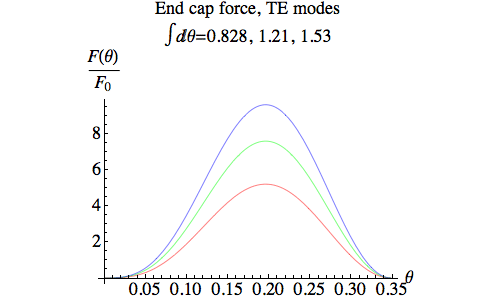Numerical data for example modes, r1=2.5 cm, r2=10 cm, θw = 20° (Fz is the absolute value of either the total side wall force or the net end-cap force) TM modes: Fz / Utotal = 9.2953 N J–1 9.59222 N J–1 9.83426 N J–1 TE modes: Fz / Utotal = 9.79783 N J–1 9.79777 N J–1 9.79516 N J–1

## Currents, heating and Q factors

So far, we have treated the cavity walls as being made of a perfect conductor. In reality, of course, this will not be true, and the currents induced in the walls by the electromagnetic field will meet resistance, and dissipate energy as heat. If this rate of loss is not too great, and the energy in the cavity is constantly being replenished by a source of microwaves at the resonant frequency, then it’s reasonable to assume that the field geometry will closely resemble that of the lossless mode.

We can find the current at any point on the wall by applying the Ampère-Maxwell Law to a small square loop that surrounds an element of the wall material, with one edge just inside the cavity (parallel to the wall), one edge outside the cavity, and two edges crossing the wall to complete the loop. We align the plane of the loop so that the edge inside the cavity is parallel to the magnetic field there. The Ampère-Maxwell Law then tells us that the current enclosed by the loop, running through the wall perpendicular to the loop, will be proportional to the magnetic field just inside the cavity.

In the TM modes, the magnetic field circles around the axis, and induces currents than run along the side walls in a purely radial direction, and along the end caps towards and away from the centre of each cap. In the TE modes, the currents run around the axis.

The resistance of a given area of the wall will be constant, so the power dissipated as heat per unit area will be proportional to the square of the current, and hence proportional to the square of the magnetic field strength at the wall.

### TE modes

For the TE modes there is no electric field at the wall, so the heat production per unit area will be proportional to the energy density, and hence also to the pressure. Although we have already shown images of the energy density for a cross section through the cavity, the high peak density in the middle of the cavity meant that little detail was visible along the walls, so we will plot new images scaled according to the maxima reached on the walls: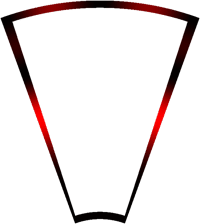Heat production per unit area for Transverse Electric (TE) modes: lowest n value, three lowest k values.

If the cavity was surrounded by vacuum, the heat produced would be lost as infrared radiation, giving rise to a back-reaction on the cavity wall. That force would be normal to the wall, so if we neglect the effects of heat conduction in the cavity wall (which could redistribute the intensity of radiation from different parts of the surface) all the calculations would be identical to those we carried out for the internal radiation pressure for the TE modes, and the force would sum to zero. Even if heat conduction destroyed this perfect balance, relativity would put an absolute ceiling of P/c (where P is power and c is the speed of light) on the thrust due to this radiation pressure; this works out at 3.33 micronewtons per kilowatt.

If the cavity is surrounded by air, heat will be lost by conduction into the air. Given the asymmetry of the cavity’s shape and the heat production rate along the wall, there is no reason for the forces due to the air flow to sum to zero.

### TM modes

For the TM modes, the rate of heat production per unit area is not proportional to any quantity we’ve previously considered, so we compute it directly from the magnetic field strength:

ΔH / ΔA = H0 [Rα(r) Q'(θ)]2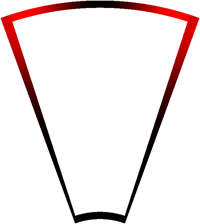Heat production per unit area for Transverse Magnetic (TM) modes: lowest n value, three lowest k values.

For a cavity surrounded by vacuum, the integrals for the radiation pressure due to radiative heat loss will no longer sum to zero. However, the ceiling of 3.33 micronewtons per kilowatt still applies.

For a cavity surrounded by air, any force experienced will again depend on the asymmetry of the air flow.

### Q factors

The Q factor of a mode in a resonant cavity gives the number of oscillations of the mode before losses reduce the energy in the cavity by a factor of exp(2π). In terms of the rate of power loss due to heating, H, the frequency of the mode, f, and the initial total energy in the cavity, Utotal:

Q = 2π f Utotal / H

To compute Q factors for our cavity, we need to look in more detail at the resistance of the cavity wall. For a high-frequency alternating current, the resistivity ρ and physical thickness of the wall material cannot be used directly to compute the resistance of a given area, because the current will be confined to a narrow surface layer rather than flowing through the entire thickness of the wall. The depth of this layer is known as the skin depth, δ, and it will depend on both the bulk resistivity of the material and the frequency of the current. Given the skin depth, the frequency-dependent surface resistivity, Rs, can then be computed:

δ = √(ρ / π f μ)
Rs = ρ / δ = √(π f μ ρ)

where μ is the permeability of the wall material, which for non-ferromagnetic metals will be very close to the permeability of free space, μ0. For copper, ρ = 1.72 × 10–8 ohm metres, and Rs = 2.61 × 10–7f ohms.

Then if ΔH / ΔA is heat production per unit area:

ΔH / ΔA = H0 B2
H0 = (1/2) Rs / μ02

The factor of 1/2 appears because we take the time-averaged value over one cycle, and the factor of 1/μ02 comes from Ampere’s Law, which relates the current in the wall to the magnetic field. We integrate ΔH / ΔA to obtain the total heat production in the cavity walls, in terms of Utotal. If a known value of microwave power, P, is being supplied to the cavity at the resonant frequency, then it will reach an equilibrium with H = P, and the ratio Utotal / H can be used to determine Utotal, the total energy content of the cavity.

 Numerical data for example modes, r1=2.5 cm, r2=10 cm, θw = 20° (These values assume an idealised smooth copper surface) TM modes: Utotal / H = 0.46 mJ/kW 0.90 mJ/kW 1.21 mJ/kW Q = 11,928 34,822 59,586 TE modes: Utotal / H = 0.72 mJ/kW 0.64 mJ/kW 0.59 mJ/kW Q = 33,753 37,864 41,252

## Proof of zero force for any shape of cavity

Despite the asymmetry of our truncated spherical cone along the z-axis, the net force from radiation pressure on its walls is zero. How can we be sure, though, that there isn’t some other shape that will yield a non-zero net force?

To see what the net force will be in a resonant cavity of a completely arbitrary shape, we need to construct the stress tensor  for the electromagnetic field. This can be defined as a three-by-three matrix T with components:

Tij = (ε0 / 2) [ (E2 + c2 B2) δij – 2 EiEj – 2 c2 BiBj ]

The subscripts i and j range from 1 to 3, and correspond to the Cartesian x, y and z coordinates. The symbol δij is the Kronecker delta, equal to 1 if i=j, and 0 otherwise.

If we have a small area whose unit normal vector is n, then the force per unit area due to the electromagnetic field in a region that n points away from is equal to Tn, where we multiply the matrix T and the vector n in the usual way. A careful analysis of any scenario involving an electromagnetic field will yield a force in agreement with this formula  (but note that there are other sign conventions in use, where T is defined to be the opposite of the matrix given here, and the force is measured across a surface element facing in the opposite direction).

Now, suppose we take one of the rows of T, and, treating it as a vector field, compute its divergence. For example, if we take the first row:

div T1 = ∂T11 / ∂x + ∂T12 / ∂y + ∂T13 / ∂z

It’s a tedious but straightforward calculation to evaluate this as:

div T1 = ε0 [ – E1 (div E) + E2 (curl E)3E3 (curl E)2c2 B1 (div B) + c2 B2 (curl B)3c2 B3 (curl B)2 ]

We get similar results for the divergence of the other rows, and we can package all three results quite compactly as:

div T = ε0 [ E × (curl E) + c2 B × (curl B) – (div E) Ec2 (div B) B ]

where “×” here indicates the vector cross product.

Maxwell’s equations tell us that div B is zero everywhere, and that in the absence of charges (as in the interior of our cavity) div E is also zero. The curls of B and E, in the absence of currents (which again holds true in the interior of our cavity), are linked to the rates of change of E and B:

c2 curl B = ∂E / ∂t
curl E = –∂B / ∂t

So we have:

div T = ε0 [ –E × (∂B / ∂t) + B × (∂E / ∂t) ]
= –ε0 ∂[E × B] / ∂t
= –(1/c2) ∂S / ∂t
where S = c2 ε0 E × B

The vector field S is known as the Poynting vector, and it describes the rate of flow of energy per unit area in an electromagnetic field. The quantity S/c2 gives the momentum per unit volume contained in the field.

If we apply Gauss’s Theorem to the integral over the walls of an arbitrarily-shaped cavity of any one of the rows of T, say Ti, we obtain:

(Net force)i = ∫wall Ti · dA = ∫interior div Ti dV = –(1/c2) ∫interior (∂Si / ∂t) dV

If the cavity contains a standing wave, then the fields will have a harmonic time dependence of the form sin(ωt) or cos(ωt), and over one complete cycle of the mode, a period of 2π/ω, all the fields will return to their origin values. So at each point in the interior of the cavity, we will have:

cycle (∂Si / ∂t) dt = Si(t0+2π/ω) – Si(t0) = 0

So, averaged over a complete cycle in the same way, each component of the net force on the wall will sum to zero.

## References

 “Fly by light” by Justin Mullins, New Scientist No. 2568, 9 September 2006, pp 30–34. The beginning of the article is online; the full text is only available to subscribers.

 “A Theory of Microwave Propulsion for Spacecraft” by Roger Shawyer (PDF file on New Scientist web site).

 “As the engine accelerates, momentum is lost by the electromagnetic wave and gained by the spacecraft, thus satisfying the conservation of momentum.” Response from Roger Shawyer in Letters column, New Scientist No. 2572, 7 October 2006, p 24. Also online.

 “Emdrive on trial”, New Scientist blog.

 “A Plea to Save New Scientist, The N-Category Café blog.

 New Scientist Reacts!”, The N-Category Café blog.

 Advanced Calculus for Applications by Francis B. Hildebrand, Prentice-Hall, Englewood Cliffs, New Jersey, 1976. Chapter 9, problem 69, p 520.

 Methods of Theoretical Physics by P.M. Morse and H. Feshbach, McGraw-Hill, New York, 1953. Chapter 13, Volume 2.

 The curl of an arbitrary vector field in spherical polar coordinates is given by:

curl (Ar er + Aθ eθ + Aφ eφ)
= 1/(r sin θ) [cos θ Aφ + sin θ ∂Aφ/∂θ – ∂Aθ/∂φ] er
+ 1/(r sin θ) [∂Ar/∂φ – sin θ (Aφ + rAφ/∂r)] eθ
+ (1/r) [Aθ + rAθ/∂r – ∂Ar/∂θ] eφ

This can be derived easily from a general formula for the curl in non-Cartesian coordinates; see Mathematical Handbook of Formulas and Tables by Murray R. Spiegel, McGraw-Hill, New York, 1968, pp 125–126.

 Gravitation by Charles Misner, Kip Thorne and John Wheeler, W.H. Freeman, San Francisco, 1973. Chapter 5, Section 5.6.

 For example, consider a charged capacitor, with two plates of area A separated by a distance d, in vacuum. If we choose coordinates so that the electric field running between the plates lies along the x-axis, then E1=E, and all other components of E and B are zero. We then have:

T11 = –(ε0 / 2) E2
T22 = T33 = (ε0 / 2) E2
All other components of T are zero.

If we compute the force on either plate, T11 tells us there is a tension of (ε0 / 2) E2, which makes sense because the opposite charges on the plates are attracting each other, and the exact tension is in agreement with the amount of work that would need to be done to move one of the plates, increasing their separation, d. On the other hand, the side walls of the capacitor are under pressure, as indicated by T22 and T33, because the energy density in the capacitor would decrease faster than the volume would increase if the area A became larger (while the plates held the same charge); in other words, work could be done by letting the capacitor expand sideways.Science Notes / Resonant Modes of a Conical Cavity / created Tuesday, 24 October 2006 / revised Tuesday, 5 August 2014